Constituency Parsing with a Self-Attentive EncoderModel F1 score Paper / Source
Self-attentive encoder + ELMo (Kitaev and Klein, 2018) 95.13 Constituency Parsing with a Self-Attentive Encoder
Model combination (Fried et al., 2017) 94.66 Improving Neural Parsing by Disentangling Model Combination and Reranking Effects
In-order (Liu and Zhang, 2017) 94.2 In-Order Transition-based Constituent Parsing
Semi-supervised LSTM-LM (Choe and Charniak, 2016) 93.8 Parsing as Language Modeling
Stack-only RNNG (Kuncoro et al., 2017) 93.6 What Do Recurrent Neural Network Grammars Learn About Syntax?
RNN Grammar (Dyer et al., 2016) 93.3 Recurrent Neural Network Grammars
Transformer (Vaswani et al., 2017) 92.7 Attention Is All You Need
Semi-supervised LSTM (Vinyals et al., 2015) 92.1 Grammar as a Foreign Language
Self-trained parser (McClosky et al., 2006) 92.1 Effective Self-Training for Parsing

# 摘要

Attention的主要想法借鉴了谷歌的神作：Attention Is All You Need，这篇论文网上也有很多讲解了，我挑选了一篇讲解比较好的，大家可以先看看：Attention Is All You Need

# 模型

## 词向量表示

• word embdding：$w_t$，这部分可以用随机初始化的向量，也可以用CharLSTM，也可以用预训练的词向量。
• tag embdding：$m_t$
• 位置向量：$p_t$

$z_t = w_t + m_t + p_t$

## Self-Attentive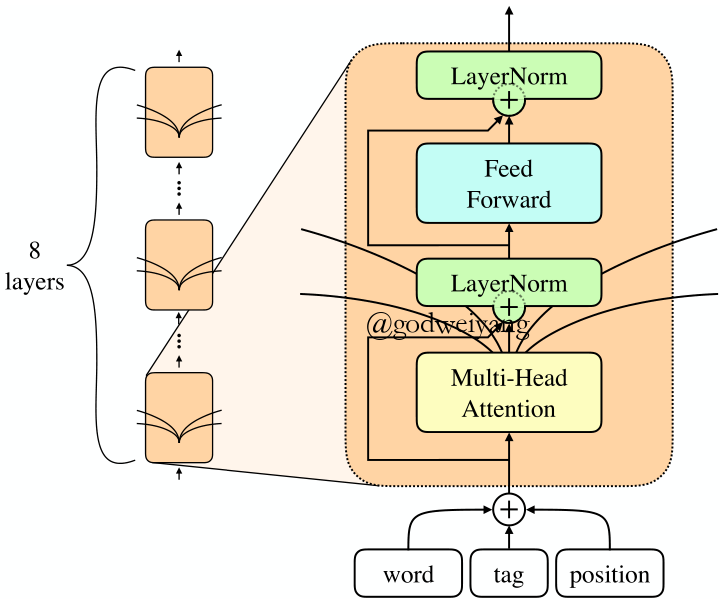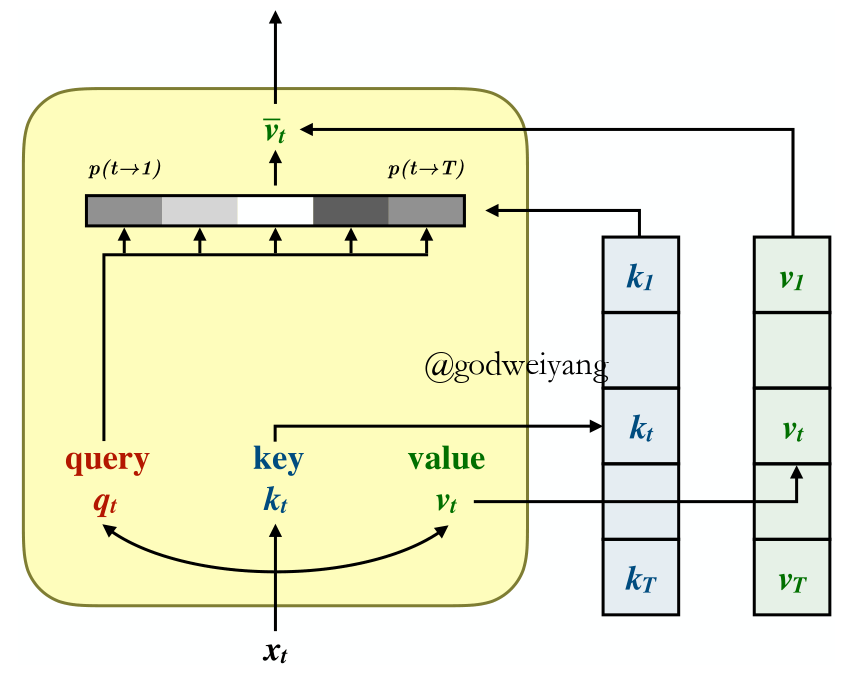${\rm{SingleHead}}(X) = \left[ { {\rm{Softmax}}\left( {\frac{ {Q{K^{\rm{T}}}}}{ {\sqrt { {d_k}} }}} \right)V} \right]{W_o}$

$p(i \to j) \propto \exp \left( {\frac{ { {q_i} \cdot {k_j}}}{ {\sqrt { {d_k}} }}} \right)$

${ {\bar v}_i} = \sum\nolimits_j {p(i \to j){v_j}}$

${\rm{MultiHead(}}X) = \sum\limits_{i = 1}^8 { {\rm{SingleHea}}{ {\rm{d}}^{(i)}}(X)}$

${\rm{FeedForward}}(x) = {W_2}{\rm{relu}}({W_1}x + {b_1}) + {b_2}$

## Span得分

$s(i,j, \cdot ) = {M_2}{\rm{relu}}({\rm{LayerNorm}}({M_1}v + {c_1})) + {c_2}$

$v = [{ {\vec y}_j} - { {\vec y}_i},{ {\mathord{\buildrel{\lower3pt\hbox{\scriptscriptstyle\leftarrow}} \over y} }_{j + 1}} - { {\mathord{\buildrel{\lower3pt\hbox{\scriptscriptstyle\leftarrow}} \over y} }_{i + 1}}]$

# 模型分析

## Content vs Position Attention

$z_t=[w_t+m_t;p_t]$

$QK^{\rm{T}} = {Q_c}K_c^{\rm{T}} + {Q_p}K_p^{\rm{T}}$

$W = \left[ {\begin{array}{*{20}{c}}{ {W_c}}&0\\0&{ {W_p}}\end{array}} \right]$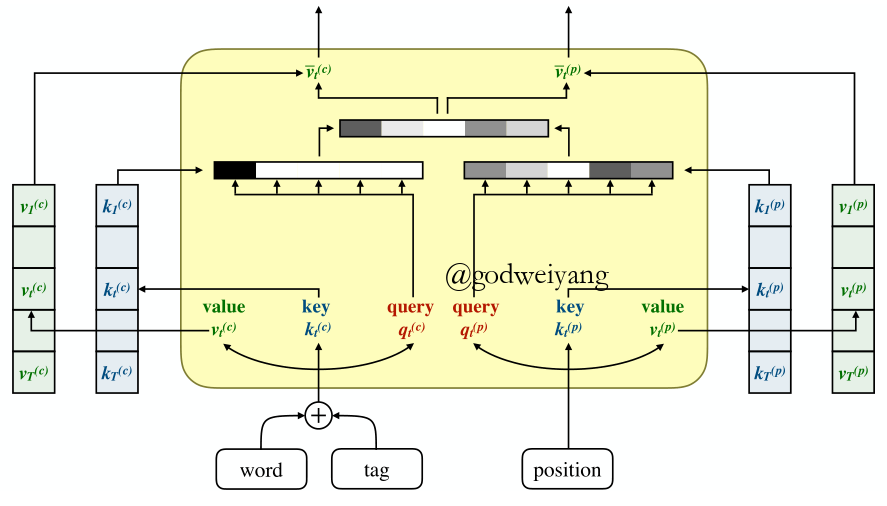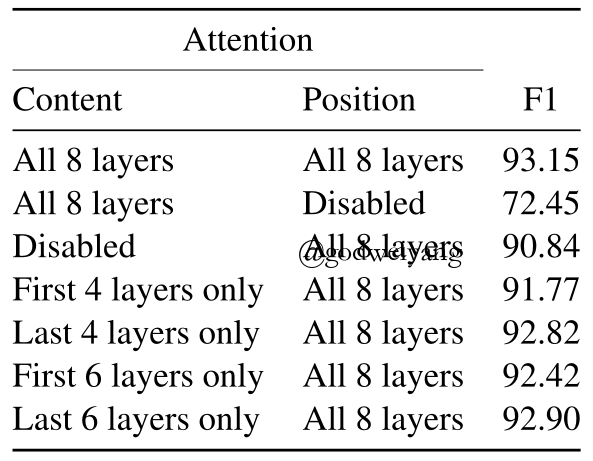## 窗口Attention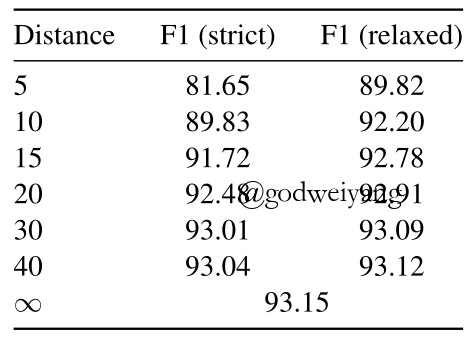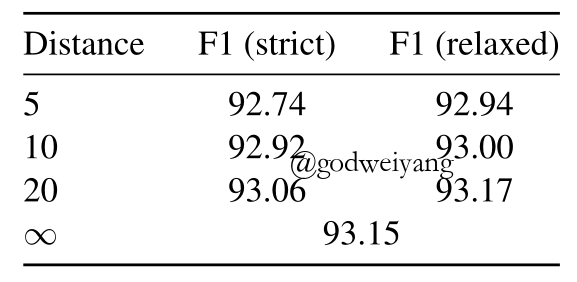# 模型的一些改进

## Subword Features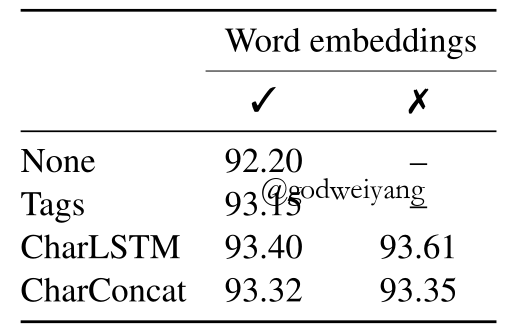## 外部词向量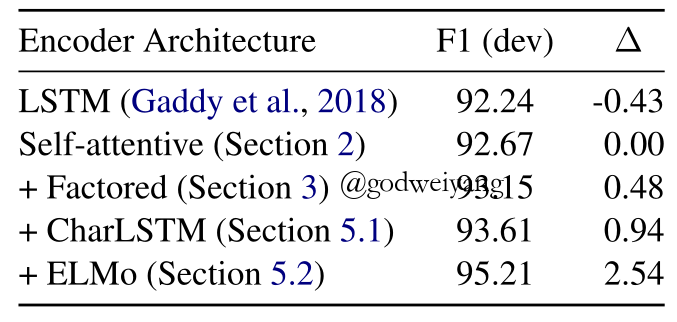F1值大大提高，达到了惊人的95.21%。

# 实验结果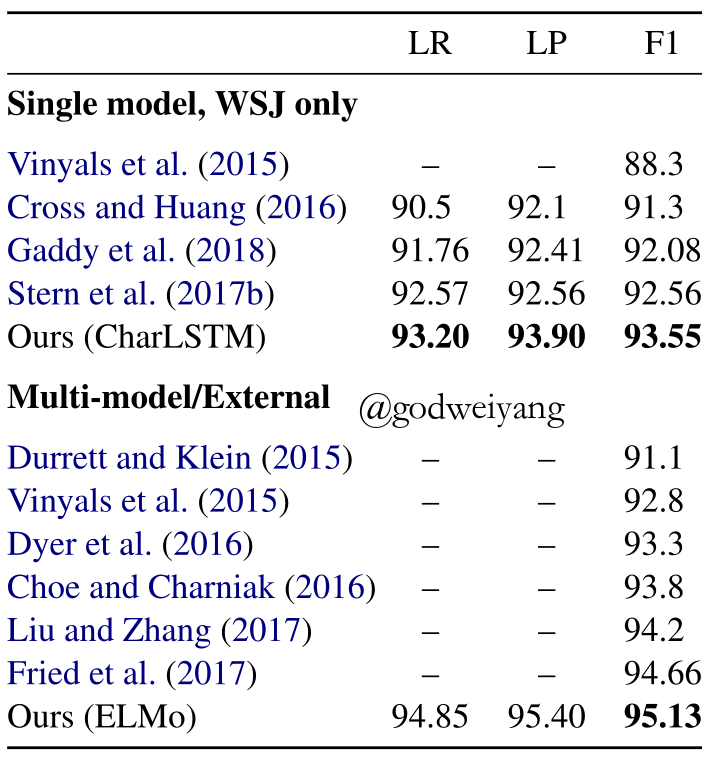# 总结

• subword的信息（CharLSTM）和预训练的词向量非常重要。
• 将content和position信息分开可以提升实验结果。

转载规则

《Constituency Parsing with a Self-Attentive Encoder》 韦阳 采用 知识共享署名 4.0 国际许可协议 进行许可。
上一篇Straight to the Tree： Constituency Parsing with Neural Syntactic Distance

2018-07-19What's Going On in Neural Constituency Parsers? An Analysis

2018-07-03
目录